# GSEB Solutions Class 7 Maths Chapter 13 Exponents and Powers InText Questions

Gujarat Board GSEB Textbook Solutions Class 7 Maths Chapter 13 Exponents and Powers InText Questions and Answers.

## Gujarat Board Textbook Solutions Class 7 Maths Chapter 13 Exponents and Powers InText QuestionsTry These (Page 250)

Question 1.
Find five more such examples, where a number is expressed in exponential form. Also identify the base and the exponent in each case.
Solution: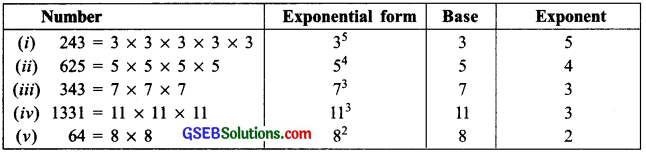Note:
1. x × x × x × x = x4 is read as ‘x raised to the power 4’ or ‘4th power of x’.
2. x²y5 is read as ‘x squared into y raised to power 5’.
3. p6q3 is read as ‘p raised to the power 6 into q cubed’.Try These (Page 251)

Question 1.
Express:
(i) 729 as a power of 3
(ii) 128 as a power of 2
(iii) 343 as a power of 7
Solution:
(i) 729
We have:
729 = 3 x 3 x 3 x 3 x 3 x 3 = 36
Thus, 729 = 36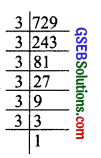(ii) 128
We have:
128 = 2 x 2 x 2 x 2 x 2 x 2 x 2 = 27
Thus, 128 = 27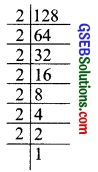(iii) 343
We have:
343 = 7 x 7 x 7 = 73
Thus 343 = 73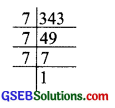Try These (Page 254)

Question 1.
Simplify and write in exponential form:
(i) 25 x 23
(ii) p3 x p2
(iii) 4³ x 4²
(iv) a3 x a2 x a7
(v) 53 x 57 x 512
(vi) (-4)100 x (4)20
Solution:
Since am x an = am+n, therefore:
(i) 25 x 23:
We have: 25 x 23 = 25+3 = 28

(ii) p3 x p2:
We have: p3 x p2 = p3+2 = p5

(iii) 43 x 4²:
We have: 4³ x 4² = 43+2 = 45

(iv) a3 x a2 x a7
We have: a3 x a2 x a7 = a3+2+7 = a12

(v) 53 x 57 x 512
We have: 53 x 57 x 512 = 53+7+12 = 522

(vi) (-4)100 x (-4)20
We have: (-4)100 x (-4)20 = (-4)100+20 = (4)120

Note: The above rule is possible only for same bases. It is not true for different bases. Thus, 23 x 3² will not obey this rule.Try These (Page 255)

Question 1.
Simplify and write in exponential form: (example 116 – 112 = 114 )
(i) 29÷ 23
(ii) 108 ÷ 104
(iii) 911 ÷ 97
(iv) 2015 ÷ 2013
(v) 713 ÷ 710
Solution:
Since am ÷ an = am-n, therefore;
(i) 29÷ 23:
We have: 29 ÷ 23 = 29-3 = 26

(ii) 108 ÷ 104:
We have: 108 ÷ 104 = 108-4 = 104

(iii) 911 ÷ 97
We have: 911 ÷ 97 = 911-7 = 94

(iv) 2015 ÷ 2013:
We have: 2015 ÷ 2013 = 2015-13 = 202

(v) 713 ÷ 710:
We have: 713 ÷ 710 = 713-10 = 73

Try These (Page 255)

Question 1.
Simplify and write the answer in exponential form:
(i) (62)4
(ii) (2²)100
(iii) (750
(iv) (53)7
Solution:
Since (am)n = am×n = amn, therefore;
(i) (62)4:
We have: (62)4 = 62×4 = 68

(ii) (2²)100:
We have: (2²)100 = 2² x 100 = 2200

(iii) 750)²:
We have: (750)² = 750×2 = 7100

(iv) (53)7:
We have: (53)7 = 53×7 = 521Try These (Page 256)

Question 1.
Put into another form using am x bm = (ab)m:
(i) 43 x 23
(ii) 25 x b5
(iii) a2 x t2
(iv) 56 x (-2)6
(v) (-2)4 x (-3)4
Solution:
(i) 43 x 23:
We have: 43 x 23 = (4 x 2)3 = 83

(ii) 25 x b5:
We have: 25 x b5 = (2 x b)5 = (2b)5

(iii) a2 x t2:
We have: a2 x t2 = (a x t)2 = (at)2

(iv) 56 x (-2)6:
We have: 56 x (-2)6 = [5 x (-2)]6 = (-10)6

(v) (-2)4 x (-3)4:
We have: (-2)4 x (-3)4 = [(-2) x (-3)]4 = 4Try These (Page 257)

Question 1.
Put into another form using am ÷ bn = ( $$\frac { a }{ b }$$ )m.
(i) 45 ÷ 35
(ii) 25 ÷ b5
(iii) (-2)3 ÷ b3
(iv) p4 ÷ q4
(v) 56 ÷ (-2)6
Solution: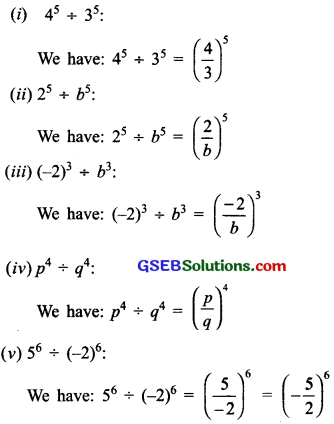Try These (Page 261)

Question 1.
Expand by expressing powers of 10 in the exponential form:
(i) 172
(ii) 5,643
(iii) 56,439
(iv) 1,76,428
Solution:
(i) 172:
We have:
172 = (1 x 100) + (7 x 10) + (2 x 1)
= 1 x 10² + 7 x 101 + 2 x 100 (∵ 10° = 1)

(ii) 5,643:
We have:
5,643 = 5 x 1000 + 6 x 100 + 4 x 10 + 3 x 1
= 5 x 103 + 6 x 102 + 4 x 101 + 3 x 100

(iii) 56,439:
We have:
56,439 = 5 x 10000 + 6 x 1000 + 4 x 100 + 3 x 10 + 9 x 1
= 5 x 104 + 6 x 103 + 4 x 102 + 3 x 101 + 9 x 100

(iv) 1,76,428:
We have:
1,76,428 = 1,00,000 + 7 x 10,000 + 6 x 1000 + 4 x 100 + 2 x 10 + 8 x 1
= 1 x 105 + 7 x 104 + 6 x 103 + 4 x 102 + 2 x 101 + 8 x 100